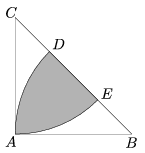Mathematical and Physical Journal
for High Schools
Issued by the MATFUND Foundation
 Already signed up? New to KöMaL?

#Problem K. 195. (January 2009)

K. 195. The isosceles triangle ABC has a right angle at A; the length of its legs is AB=AC=4 cm. The circles with centers at B and C of radius 4 cm intersect the hypotenuse at D and E, respectively. What is the area of the shaded domain? (See the Figure.)(6 pont)

Deadline expired on February 10, 2009.

Sorry, the solution is available only in Hungarian. Google translation

Megoldás. A satírozott területet jelölje T. Ezen kívül keletkezik még két egybevágó alakzat, ezek területe felírható:.

Így a terület:### Statistics:

 170 students sent a solution. 6 points: 55 students. 5 points: 50 students. 4 points: 30 students. 3 points: 12 students. 2 points: 7 students. 1 point: 1 student. 0 point: 8 students. Unfair, not evaluated: 7 solutionss.

Problems in Mathematics of KöMaL, January 2009